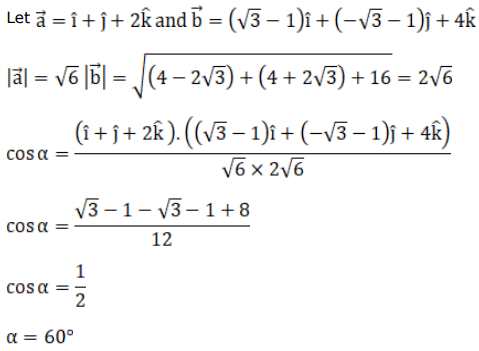# The angle between two lines having direction ratios

Question:

The angle between two lines having direction ratios $1,1,2$ and $(\sqrt{3}-1),(-\sqrt{3}-1), 4$ is

A. $\frac{\pi}{6}$

B. [] $\frac{\pi}{2}$

C. $\frac{\pi}{3}$

D. $\frac{\pi}{4}$

Solution: